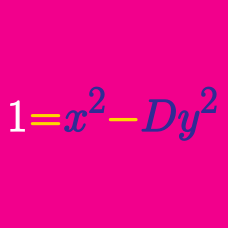Number Theory

# Quadratic Diophantine Equations: Level 3 Challenges

$\large \color{#3D99F6}{x}^2-\color{#D61F06}{y}^2=2011$

How many integral solutions $(\color{#3D99F6}{x},\color{#D61F06}{y})$ are there for the equation above?

\begin{aligned} ab+cd&=&38 \\ ac+bd&=&34\\ ad+bc&=&43\\ \end{aligned}

Let $a,b,c$ and $d$ positive integers such that they satisfy the system of equations above. Find $a+b+c+d$.

Find the number of ordered quadruples of positive integers $(x,y,p,q)$ satisfying

$x^3y-xy^3=pq,$

and $p,q$ are prime numbers.

This problem is posed by Daniel C.

$\large \frac{1}{x}+\frac{1}{y}=\frac{1}{156}$

Find the number of ordered pairs of positive integers $(x, y)$ that satisfy the equation above.

How many ordered tuples of natural numbers $(a,b,c)$ satisfy $\frac{1}{a}+\frac{1}{ab}+\frac{1}{abc}=1?$

×